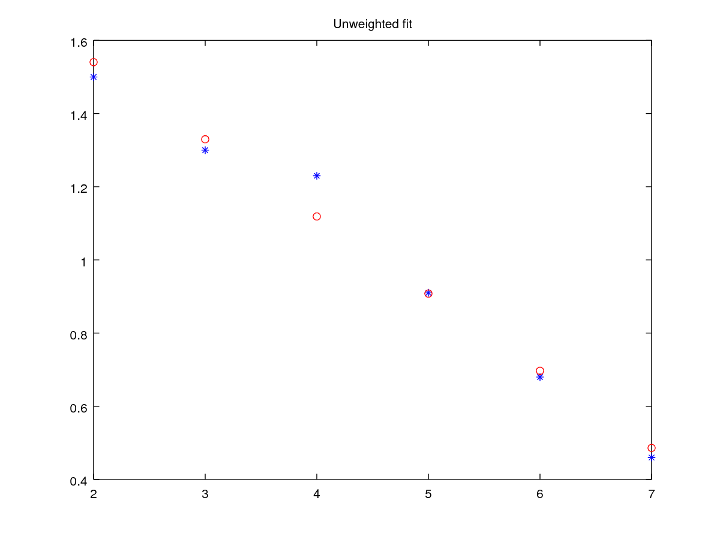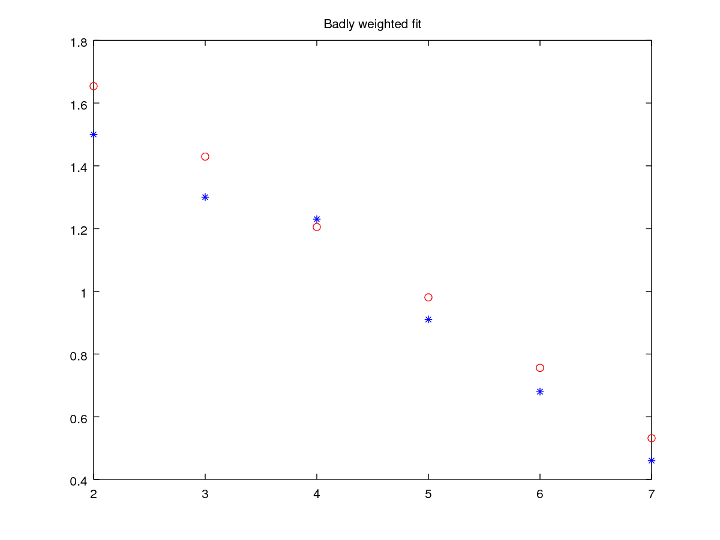# Scaling, Weighting, and Least Squares

Developing Models for Kalman Filters

Previous installments examined how to build the Normal Equations to find an optimal least squares estimate for parameters defining a linear model. The word optimal is a slippery one, since the results you get depend on the data you give it and what you seek to optimize.

## Column scaling

Suppose that you have the constraint equation system for a Linear Least Squares problem, solve the Normal Equations to calculate the least squares best fit, and record the results. Now multiply one column of the constraint equations matrix by some nonzero column scaling factor. Rebuild the normal equations and solve for the "best fit" parameter solution again. What happens?

You will find that all of the parameter values will turn out the same as before, except for the one associated with the scaled column. That parameter will have its previous value divided by the scaling term that was applied to the column. If you think about this, it is the logical outcome. The scaling on the parameter balances out the scaling on the column of data, so that the "optimal" result remains unchanged.

This is the situation that arises when you decide to change physical units for some of the measurements. It makes sense that this would not fundamentally alter the general nature of the solution. However, it is probably a good idea to keep the sizes of the terms in all columns roughly the same size to avoid numerical "loss of precision" problems.

## Row weighting

A row scaling or weighting operation is not quite so obvious.

Consider the `k`-th equation in an overdetermined system of constraint equations, with known input values `m` and unknown model parameters ` a`, ` b`, and ` c`. The corresponding output value should be ` y`. The `k`-th constraint row before modification is:

`    mk1·a +  mk2·b +  mk3·c =  yk`

Starting from this relationship, apply a positive weighting factor `W` to every term on both sides of the expression.

```    W mk1·a + W mk2·b + W mk3·c =  W yk
```

At first, it seems like this changes nothing: it is an algebraic fact that the constraint equations before and after scaling are mathematically equivalent. However, there is a surprising and important impact on the results of the least squares fitting problem. To see this, let's take a concrete example.

Suppose the goal it to fit a best first-order polynomial model to the following data set.

constant input x input y output
1 2.0 1.50
1 3.0 1.30
1 4.0 1.18
1 5.0 0.92
1 6.0 0.69
1 7.0 0.46

The following compares the best-fit line and the values from the constraint table.```BEST FIT: (original problem)
Pfit =
1.94562
-0.20829```

We can see that the actual value of output `y` for the third row, corresponding to the input value ` x = 4`, lies well away from the best-fit line. This could indicate a nonlinear behavior of the actual system, or perhaps it is just an unusually large measurement error. The fit error at that point is more than twice the largest fit error at any other location. In trying to match this "outlier" point, the fit becomes worse at all the other points.

```     RESIDUAL ERRORS OF FIT:
1   -0.0290476
2   -0.0207619
3    0.0675238
4    0.0158095
5   -0.0059048
6   -0.0276190
```

Now apply row scaling to that third constraint row, using a scaling factor of 10. Apply this factor both to the matrix row and the term in the right-hand-side column. The modified terms for the third row become:

constant input x input output
10 40 11.8

Construct the normal equations again, with the adjusted terms replacing the original ones in the third row. Solve to obtain the new best fit. Check the new results against the original data set.

```BEST FIT: (row-scaled problem)
Pfit =
2.05573
-0.21894

NEW RESIDUAL ERRORS OF FIT:
Resid =
1   -0.1178457
2   -0.0989042
3    0.0037299
4   -0.0410212
5   -0.0520797
6   -0.0631383
```It is clear that this set of best fit parameters is different. The "best fit" curve is dramatically closer to that peculiar third point, while the fit errors at all of the other points are significantly worse.

When you think about what the Least Squares problem is trying to accomplish, this result is reasonable. A Least Squares optimization is trying to keep the combined squared error effects as small as possible. To do that, it needs to satisfy the scaled constraint equation much more closely than the rest, because if it does not, the amplified coefficient values would cause a correspondingly amplified contribution to the squared-error sum.

It seems perverse that we can have two constraint systems, where algebraically the constraint equations are completely equivalent, yet they have different optimal solutions. Well, when you see that word "optimal," you have to ask the question "When optimizing what?"

## A mathematical representation

Row scaling can be applied to all rows as easily as one.

Mathematically, a row scaling operation can be represented by a matrix `W` that is:

1. square
2. has strictly positive terms along its main diagonal
3. has zero terms in all other matrix locations.

Applying this scaling matrix to multiply both sides of a Linear Least Squares equation system changes it into a new problem in which each row has a row-scaling factor applied.

```   M · P  =  Y    becomes    W M · P  =  W Y
```

You can think of an ordinary Linear Least Squares equation system as being a special case in which the weighting matrix `W` is chosen to be an identity matrix.

The corresponding Normal Equation condition for the "least squares best fit" can be set up and solved for the modified problem.

```   (W M)T  · W M · P = (W M)T · (W Y)

MT W2 M · P =  MT W2 · Y

P =  (MT  W2 M)-1 · (MT W2) · Y
```

Because of the large number of rows in a typical Least Squares fitting problem, building the weighting matrix explicitly is massively inefficient. Scaling factors are typically incorporated directly into each row as the Least Squares problem constraint matrix is constructed.

## Interpretations

As we have seen, the meaning of "best-fit" is distorted by the row scaling. It seems hard to imagine why you would ever want to do such a thing, considering the potential hazards. However, when used with care, weighting can be very useful.

• One interpretation of the `W` matrix weights is "trustworthiness." When the information in the corresponding `k`-th constraint is more reliable, a larger `Wkk` term in the weighting matrix tells the least squares solver to put more emphasis on satisfying it. A smaller `Wkk` term in the weighting matrix tells the solver to accept a relatively large fit error. Remember that "outlier" term in the numerical example? If a scale factor of 1/4 had been used for that oddball term, instead of a scale factor of 10, the fit would have improved at every other point.• Another interpretation of the `W` matrix weights is "relevance." Suppose the constraint matrix is being updated to insert new information about current conditions. Is the new information more relevant than information observed 10000 samples in the past, or 10000000 samples in the past?

## Coming next

We will follow up by examining a systematic row weighting strategy that makes Least Squares methods useful for adaptive parameter adjustments, responsive to current conditions. This will combine things covered in this installment and the previous one about sequential updates.

Contents of the "Developing Models for Kalman Filters" section of the ridgerat-tech.us website, including this page, are licensed under a Creative Commons Attribution-ShareAlike 4.0 International License unless otherwise specifically noted. For complete information about the terms of this license, see http://creativecommons.org/licenses/by-sa/4.0/. The license allows usage and derivative works for individual or commercial purposes under certain restrictions. For permissions beyond the scope of this license, see the contact information page for this site.